## 25 February 2014

An expression having one or more variables is called an algebraic expression.

An algebraic expression may or may not contain mathematical operators like the symbols of addition, subtraction and multiplication.

The quantities in an algebraic expression - variable,constant or combinations of both, joined by plus or minus signs, are called its terms
The elements whose product forms a term of an algebraic expression are called the factors of that term.  The numerical factor of a term containing a variable is called the coefficient of the term.Algebraic expressions that contain only one term are called monomials. Algebraic expressions that contain only two terms are called binomials. Algebraic expressions that contain only three terms are called trinomials.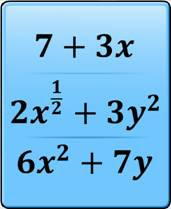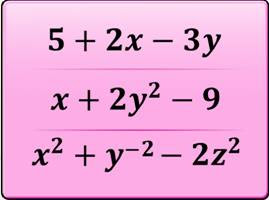Algebraic expressions with all variables having whole number exponents or powers are called polynomials. The expressions in which the powers of variables are negative or rational numbers are not polynomials.
Terms that have the same power of the same variable are called like terms.  Terms that do not contain the same power of the same variable are called unlike terms.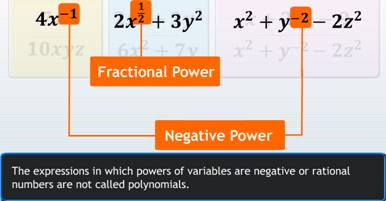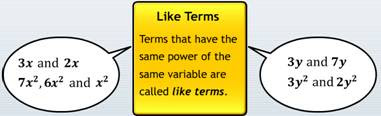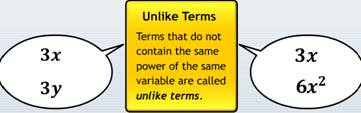To add or subtract algebraic expressions:
• Arrange the expressions in separate rows, so that all like terms are one below the other and all unlike terms are in separate columns.
• Write all terms with their correct positive or negative signs.
• While subtracting, keep in mind how the minus sign between the expressions changes the signs of the terms of the expression being subtracted.

 With our free PDF notes you can get success in any competitive or entrance exams like CTET,  KVS, NET, CAT, MAT, CMAT, SSC, B.ED, IBPS Recruitment, IAS, CSAT, State Civil Services Exams, UPTET, PSTET, HTET & many more. It also provides NCERT solutions, CBSE, NTSE, Olympiad study material, Indian General Knowledge, English, Hindi, Mathematics, Current affairs, Science, S.ST, model test papers, important Questions and Answers asked in CBSE examinations.